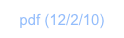Constants & UnitsND1 has some built-in constants. You can use them by name on the edit line or in expressions, just like variables. Unlike variables, you cannot assign a value to them.

In addition to the constants listed here, there’s a Constants downloadable folder, which contains many physical constants. These can be installed into the calculator for global availability.

ND1 features an extensive unit conversion system. Its use is described in Just a Few More Things. You can refer to a unit name by either its long name (e.g., “Inch”), or its short name (e.g., “in”), as indicated in the list below.

The units appear in the Units section of My Data, and are fully editable: you can add, or remove, individual units as well as unit categories. Unit conversions are specified either by factor or RPL program (see Temperature below).

Overview

version             the current version number of the calculator / MorphEngine (presently, “1.1”)

e                        the number e (JavaScript value Math.E)

i                         the imaginary number i, i.e., (0,1)

ln10                   the natural logarithm of 10 (JavaScript value Math.LN10)

ln2                     the natural logarithm of 10 (JavaScript value Math.LN2)

π, pi                  the number pi (JavaScript value Math.PI)

half_pi_period two divided by pi

MAXR                the largest positive number representable with non-big numbers (JS value Number.MAX_VALUE)

MINR                 the smallest positive number representable with non-big numbers (JS value Number.MIN_VALUE)

MEM                  the amount of memory available (10 MB on iPhone and iPod touch)

sqrt2                 the square root of two (JavaScript value Math.SQRT2)

ConstantsWeight [g]:

Short Ton [ton]                   907184.74

Grain [ga]                           0.06479891

Troy Pound [lbt]                 373.2417

Slug [slug]                          14593.9029372

Carat [ct]                            0.2

Ounce [oz]                         28.349523125

Atomic Mass [u]                 1.66057e-24

Troy Ounce [ozt]                31.103475

Metric Ton [t]                      1000000

Long Ton [tonUK]               1016046.9088

Pound [lb]                           453.59237

Length [m]:

Astro Unit [au]                    149597900000

Parsec [pc]                         30856781858500000

Foot [ft]                               0.3048

Inch [in]                               0.0254

Rod [rod]                             5.02921005842

Chain [chain]                       20.1168402337

Statute Mile [miUS]             1609.34721869

Angstrom [Å]                       1e-10

Fermi [fermi]                       1e-15

Mile [mi]                              1609.344

Yard [yd]                              0.9144

Nautical Mile [nmi]               1852

Mil [mil]                                 0.0000254

Survey Foot [ftUS]               0.304800609601

Light Year [lyr]                      9460528404880000

Fathom [fath]                        1.82880365761

Area [m²]:

Square Foot [sqft]                 0.09290304

Acre [acre]                            4046.87260987

Barn [b]                                 1e-28

Are [a]                                   100

m2 [m^2]                               1

Volume [m³]:

m3 [m^3]                                1

U.S. Gallon [gal]                     0.003785411784

Pint [pt]                                   0.000473176473

U.K. Fluid Ounce [ozUK]        0.000028413075

U.S. Fluid Ounce [ozfl]           0.0000295735295625

Peck [pk]                                0.0088097675

Stere [st]                                1

U.K. Gallon [galUK]                0.004546092

Bushel [bu]                             0.03523907

Liter [l]                                    0.001

Barrel [bbl]                              0.158987294928

Cup [cu]                                  0.000236588265

Board Ft [fbm]                         0.002359737216

Tablespoon [tbsp]                   0.0000147867647813

Teaspoon [tsp]                        0.00000492892159375

Quart [qt]                                 0.000946352946

Time [s]:

Tropical Year [yr]                    31556925.9747

Minute [min]                            60

Day [d]                                    86400

Hour [h]                                   3600

Frequency [1/s]:

Becquerel [Bq]                     1

Curie [Ci]                              37000000000

Hertz [Hz]                             1

Speed [m/s]:

Knot [knot]                              0.514444444444

Kilometers Per Hour [kph]      0.277777777777778

Miles Per Hour [mph]             0.44704

Speed of Light [c]                   299792458

Acceleration [m/s²]:

Std Freefall [ga]                      9.80665

Pressure [kg/m*s²]:

Inches of Water [inH2O]            248.84

Pascal [Pa]                                1

Pounds per Square Inch [psi]    6894.75729317

Bar [bar]                                    100000

Millimeters of Mercury [mmHg] 133.322368421

Standard Atmosphere [atm]      101325

Torr [torr]                                   133.322368421

Inches of Mercury [inHg]           3386.38815789

Force [kg*m/s²]:

Poundal [pdl]                     0.138254954376

Newton [N]                        1

Kip [kip]                             4448.22161526

Dyne [dyn]                         0.00001

Pound-Force [lbf]              4.44822161526

Gram-Force [gf]                 0.00980665

Energy [kg*m²/s²]:

Joule [J]                            1

Calorie [cal]                      4.1868

IST [Btu]                           1055.05585262

EEC [therm]                      105506000

Electron Volt [eV]              1.60219e-19

Erg [erg]                            1e-7

Power [kg*m²/s³]:

Kilo-Watt [kW]                    1000

Watt [W]                             1

Horsepower [hp]                 745.699871582

Charge [A*s]:

Coulomb [C]                        1

Temperature [K]:

Kelvin [K]                             1

Fahrenheit [°F]                     from: ≪ 32 - 5 * 9 / 273.15 + ≫ ; to: ≪ 273.15 - 9 * 5 / 32 + ≫

Celsius [°C]                          from: ≪ 273.15 + ≫ ; to: ≪ 273.15 - ≫

Angle [°]:

Degree [degree]                   0.002777777777777778